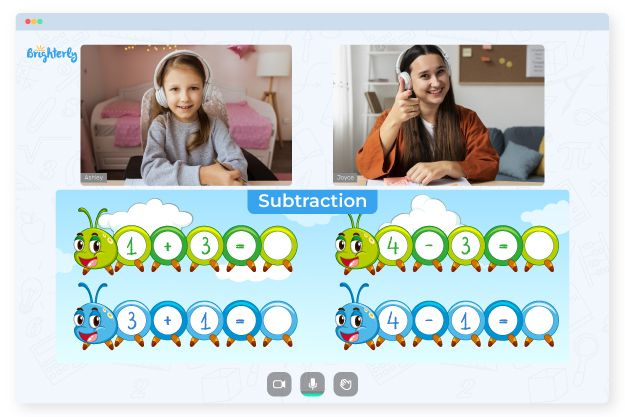# 2 Digit Subtraction With Regrouping Worksheets

Tackling double digit subtraction without regrouping worksheets can be a challenge for elementary school kids. That is why every teacher should get these tools to facilitate their students’ learning. The worksheets have exercises that help students improve their 2-digit subtraction skills, exercise their memories, and make them less scared of math problems.

## All about 2 Digit Subtraction with Regrouping Worksheets 2nd Grade Students Use

The 2 digit subtraction worksheets 2nd grade students use teach kids to subtract by showing them the right place to put the subtrahend and minuend in the column using their position, unit, and value.

Math for Kids

Is Your Child Struggling With Math?
1:1 Online Math TutoringThese two digit subtraction with regrouping worksheets provide an accurate set of problems that students can solve at their own pace. They also include answers to cross check and confirm if the solutions are in line with what is required. Many teachers are integrating worksheets in their lesson plans, and if you have students or kids who need extra guidance to master 2-digit subtraction you should give a two digits subtraction with regrouping worksheet a try too.### 2 Digit Subtraction With Regrouping Worksheets PDF

Two Digits Subtraction With Regrouping Worksheet### 2 Digit Subtraction With Regrouping Worksheets PDF

Double Digit Subtraction Without Regrouping Worksheets### 2 Digit Subtraction With Regrouping Worksheets PDF

Double Digit Subtraction Worksheets### 2 Digit Subtraction With Regrouping Worksheets PDF

2 Digit Subtraction Worksheets

### More Worksheets

Problems with Subtraction?• Does your child have difficulties with subtraction?
• Try learning with an online tutor.

Is your child struggling to understand subtraction? Start studying with an online tutor.